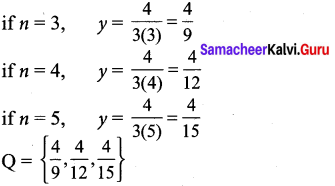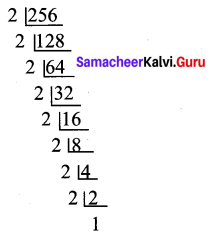## Tamilnadu Samacheer Kalvi 9th Maths Solutions Chapter 1 Set Language Ex 1.2

9th Maths Exercise 1.2 Samacheer Kalvi Question 1.
Find the cardinal number of the following sets.
(i) M = {p, q, r, s, t, u}
(ii) P = {x : x = 3n + 2, n ∈ W and x < 15}
(iii) Q = {v : v = $$\frac { 4 }{ 3n }$$ ,n ∈ N and 2 < n ≤ 5}
(iv) R = {x : x is an integers, x ∈ Z and -5 ≤ x < 5}
(v) S = The set of all leap years between 1882 and 1906.
Solution:
(i) n(M) = 6
(ii) W = {0, 1, 2, 3, ……. }
if n = 0, x = 3(0) + 2 = 2
if n = 1, x = 3(1) + 2 = 5
if n = 2, x = 3(2) + 2 = 8
if n = 3, x = 3(3)+ 2 =11
if n = 4, x = 3(4) + 2=14
∴ P= {2, 5, 8, 11, 14}
n(P) = 5

(iii) N = {1,2, 3, 4, …..}
n ∈ {3, 4, 5}n(Q) = 3

(iv) x ∈ z
R = {-5, – 4, -3, -2, -1, 0, 1, 2, 3, 4}
n(R)= 10.

(v) S = {1884, 1888, 1892, 1896, 1904}
n (S) = 5.

9th Maths Set Language Exercise 1.2 Question 2.
Identify the following sets as finite or infinite.
(i) X = The set of all districts in Tamilnadu.
(ii) Y = The set of all straight lines passing through a point.
(iii) A = {x : x ∈ Z and x < 5}
(iv) B = {x : x2 – 5x + 6 = 0, x ∈ N}
Solution:
(i) Finite set
(ii) Infinite set
(iii) A = { ……. , -2, -1, 0, 1, 2, 3, 4}
∴ Infinite set

(iv) x2 – 5x + 6 = 0
(x – 3) (x – 2) = 0
B = {3, 2}
∴ Finite set.

9th Maths Exercise 1.2 Question 3.
Which of the following sets are equivalent or unequal or equal sets?
(i) A = The set of vowels in the English alphabets.
B = The set of all letters in the word “VOWEL”
(ii) C = {2, 3, 4, 5}
D = {x : x ∈ W, 1 < x < 5}
(iii) X = A = { x : x is a letter in the word “LIFE”}
Y = {F, I, L, E}
(iv) G = {x : x is a prime number and 3 < x < 23}
H = {x : x is a divisor of 18}
Solution:
(i) A = {a, e, i, o, u}
B = {V, O,W, E, L}
The sets A and B contain the same number of elements.
∴ Equivalent sets

(ii) C ={2, 3, 4, 5}
D = {2, 3, 4}
∴ Unequal sets

(iii) X = {L, I, F, E}
Y = {F, I, L, E}
The sets X and Y contain the exactly the same elements.
∴ Equal sets.

(iv) G = {5, 7, 11, 13, 17, 19}
H = {1, 2, 3, 6, 9, 18}
∴ Equivalent sets.

9th Maths Set Language Exercise 1.2 Solutions Question 4.
Identify the following sets as null set or singleton set.
(i) A = (x : x ∈ N, 1 < x < 2}
(ii) B = The set of all even natural numbers which are not divisible by 2.
(iii) C = {0}
(iv) D = The set of all triangles having four sides.
Solution:
(i) A = { } ∵ There is no element in between 1 and 2 in Natural numbers.
∴ Null set

(ii) B = { } ∵ All even natural numbers are divisible by 2.
∴ B is Null set

(iii) C = {0}
∴ Singleton set

(iv) D = { }
∵ No triangle has four sides.
∴ D is a Null set.

9th Maths Exercise 1.2 In Tamil Question 5.
State which pairs of sets are disjoint or overlapping?
(i) A = {f, i, a, s} and B = {a, n, f, h, s)
(ii) C = {x : x is a prime number, x > 2} and D = {x : x is an even prime number}
(iii) E = {x: x is a factor of 24} and F = {x : x is a multiple of 3, x < 30}
Solution:
(i) A = {f, i, a, s}
B = {a, n, f, h, s}
A ∩ B ={f, i, a, s} ∩ {a, n,f h, s} = {f, a, s}
Since A ∩ B ≠ ϕ , A and B are overlapping sets.

(ii) C = {3, 5, 7, 11, ……}
D = {2}
C ∩ D = {3, 5, 7, 11, …… } ∩ {2} = { }
Since C ∩ D = Ø, C and D are disjoint sets.

(iii) E = {1, 2, 3, 4, 6, 8, 12, 24}
F = {3, 6, 9, 12, 15, 18, 21, 24, 27}
E ∩ F = {1, 2, 3, 4, 6, 8, 12, 24} ∩ {3, 6, 9, 12, 15, 18, 21, 24, 27}
= {3, 6, 12, 24}
Since E ∩ F ≠ ϕ, E and F are overlapping sets.

9th Standard Maths Exercise 1.2 In Tamil Question 6.
If S = {square,rectangle,circle,rhombus,triangle}, list the elements of the following subset of S.
(i) The set of shapes which have 4 equal sides.
(ii) The set of shapes which have radius.
(iii) The set of shapes in which the sum of all interior angles is 180°
(iv) The set of shapes which have 5 sides.
Solution:
(i) {Square, Rhombus}
(ii) {Circle}
(iii) {Triangle}
(iv) Null set.

9th Standard Maths Exercise 1.2 Question 7.
If A = {a, {a, b}}, write all the subsets of A.
Solution:
A= {a, {a, b}} subsets of A are { } {a}, {a, b}, {a, {a, b}}.

9th Std Maths Exercise 1.2 Question 8.
Write down the power set of the following sets.
(i) A = {a, b}
(ii) B = {1, 2, 3}
(iii) D = {p, q, r, s}
(iv) E = Ø
Solution:
(i) The subsets of A are Ø, {a}, {b}, {a, b}
The power set of A
P(A ) = {Ø, {a}, {b}, {a,b}}

(ii) The subsets of B are ϕ, {1}, {2}, {3}, {1, 2}, {2, 3}, {1, 3}, {1, 2, 3}
The power set of B
P(B) = {Ø, {1}, {2}, {3}, {1, 2}, {2, 3}, {1, 3}, {1, 2, 3}}

(iii) The subset of D are Ø, {p}, {q}, {r}, {s}, {p, q}, {p, r}, {p, s}, {q, r}, {q, s}, {r, s},{p, q, r}, {q, r, s}, {p, r, s}, {p, q, s}, {p, q, r, s}}
The power set of D
P(D) = {Ø, {p}, {q}, {r}, {s}, {p, q}, {p, r}, {p, s}, {q, r}, {q, s}, {r, s}, {p, q, r}, {q, r, s}, {p, r, s}, {p, q, s}, {p, q, r, s}

(iv) The power set of E
P(E) = { }.

9th Maths 1.2 In Tamil Question 9.
Find the number of subsets and the number of proper subsets of the following sets.
(i) W = {red,blue, yellow}
(ii) X = { x2 : x ∈ N, x2 ≤ 100}.
Solution:
(i) Given W = {red, blue, yellow}
Then n(W) = 3
The number of subsets = n[P(W)] = 23 = 8
The number of proper subsets = n[P(W)] – 1 = 23 – 1 = 8 – 1 = 7

(ii) Given X ={1,2,3, }
X2 = {1, 4, 9, 16, 25, 36, 49, 64, 81, 100}
n(X) = 10
The Number of subsets = n[P(X)] = 210 = 1024
The Number of proper subsets = n[P(X)] – 1 = 210 – 1 = 1024 – 1 = 1023.

9th Maths 1.2 Exercise Question 10.
(i) If n(A) = 4, find n[P(A)].
(ii) If n(A) = 0, find n[P(A)].
(iii) If n[P(A)] = 256, find n(A).
Solution:
(i) n( A) = 4
n[ P(A)] = 2n = 24 = 16
(ii) n(A) = 0
n[P(A)] = 20 = 1
(iii) n[P(A)] = 256n[P(A)] = 28
∴ n(A) = 8.